# Stability theory (in logic)

(diff) ← Older revision | Latest revision (diff) | Newer revision → (diff)

A branch of model theory that provides a structure theory for models of first-order theories. The collection of all first-order sentences true in a given structure forms a complete first-order theory. For example, the models of the theory of the complex fieldare exactly the algebraically closed fields of characteristic zero. Complete theories in a fixed countable languagethat have an infinite model are considered below. These theories are classified as unstable, stable, superstable, or-stable, according to the number of complete types (see below) over each model of the theory (cf. also Stable and unstable theories).

Let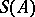denote the collection of complete types over. The cardinality ofis always at most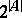, and for unstablethis maximum is usually attained.is stable in a cardinal numberif for every set of cardinality,.is unstable if it is stable in no.is stable if it is stable in some, superstable if it is stable in all cardinalities beyond the continuum and-stable if it is stable in every infinite cardinality. The increasing degrees of stability are reflected in an increasing ability to assign invariants to the models of a theory. Unstable theories include any linear ordering. Every theory of modules is stable. A separably closed field is stable, but not superstable. A superstable group does not admit an infinite descending chain of definable subgroups (with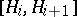infinite). For example, the additive group of integers is superstable. An algebraically closed field or an algebraic group over an algebraically closed field is-stable.

Without loss of generality all models of a first-order theorycan be considered imbedded in a fixed universal domain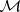. For any elementand a subsetof, the type ofover,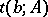, is the collection of all formulas with one free variable, and with parameters from, that are true inif the free parameters are replaced by. Two elements ofrealize the same type overprecisely if they are in the same orbit under the group of automorphisms ofthat fix.

The Löwenheim–Skolem theorem (cf. Gödel completeness theorem) guarantees that each theory has a model in each infinite cardinality. One of the principal accomplishments of stability theory has been to classify the functionsthat assign tothe number of isomorphism types of modelswith cardinality. M.D. Morley began this process with his 1963 generalization of a theorem of E. Steinitz by showing that for any first-order theory(as for the theory of algebraically closed fields),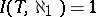(iscategorical) if and only ifis categorical in every uncountable cardinality (cf. Categoricity in cardinality). The 1971 Baldwin–Lachlan theorem continues this analogy by showing that an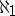categorical theory has eitherormodels.

In roughest form, each model is determined by a "skeleton" and by a "closure" of that skeleton to the entire model. The notion of a skeleton is elaborated below. The simplest type of closure is closure under functions. A first-order language can be expanded so that each subset of the universal domain has an Ehrenfeucht–Mostowski hull (generated by the functions in the language) that is a model. In general, this operation destroys stability of the theory. A weaker notion of closure assigns to eacha prime model overthat can be imbedded in every model containing. In general, no such assignment is possible, but it is ifis-stable.

S. Shelah began around 1970 the program of determining those properties of a theorythat imply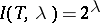for all uncountableand whose negations aid in the development of a structure theory for models of. He established that ifis unstable, or even not superstable, then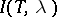has the maximum possible value,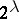, for all uncountable. Ifis unstable, there is a formula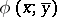that defines a linear order on an infinite set of-tuples from. The compactness theorem allows one to construct for each linear order typeof cardinalitya subset ofthat is linearly ordered byin type. By a formidable combinatorial argument, Shelah showed that forof these orderings the Ehrenfeucht–Mostowski hulls remain non-isomorphic, thus establishing that each unstable theory has the maximal number of models in each uncountable cardinality. The argument in the non-superstable case is similar, but the linear orderings are replaced by trees of height.

In any stable theory one can find a dependence relation,forks over, analogous to algebraic or linear dependence. In particular, the analogue of the exchange axiom holds. In general, however, the associated closure relation (overif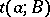forks over) is not transitive. A typeis called regular if this closure relation is transitive when restricted to the realizations of. Thus, a dimension (as in linear algebra) can be assigned to each regular type. Much of the structure theory depends on reducing the structure of a model to the structure of the regular types realized in. The collection of regular types, their dimensions and certain relations among them constitute the skeleton of a model. It may be that several regular types carry the same information regarding the structure of a model; if so, they are said to be non-orthogonal.

If each independent pair of models has a minimal closure andis superstable, then each model ofcan be analyzed as prime over a tree of countable height. If this tree is well-founded,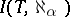can be bounded as a function of. If this closure does not exist ( "notop" ) or is not minimal ( "dop" ) or the tree is not well-founded ( "deep" ),has the maximal number of models in almost-all cardinalities. This structure/non-structure dichotomy is known as the main gap. The analysis discussed so far (1990) suffices to establish (Shelah, late 1970's) the Morley conjecture that the spectrum functionis increasing, except possibly fromto.

The exact calculation ofin the well-behaved case requires an understanding of the geometric structure of regular types. It is possible to define a combinatorial geometry on the set of realizations of a regular type. B.I. Zil'ber initiated the use of this geometric structure to obtain global information about the models of. In the simplest case (an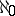-categorical strictly-minimal set) this geometry must be either trivial, an affine space over a finite field or a projective space over a finite field. With this analysis it is possible to show that no totally categorical theory is finitely axiomatizable, but every such is axiomatizable by a single sentence and an "axiom of infinity" -scheme.

R.L. Vaught conjectured in the early 1960's that a complete first-order theory has either countable many orcountable models. Shelah has established the result for-stable theories. S. Buechler and L. Newelski have extended the result to certain superstable theories. This extension depends heavily on the geometric analysis.

Stability theory interacts with classical algebra in several ways. The effect of imposing stability-theoretic constraints on algebraic structures has been extensively investigated: Every stable semi-simple ring is a matrix ring over an algebraically closed field; every superstable division ring is an algebraically closed field. G.L. Cherlin (middle of the 1970's) conjectured that every simple-stable group (of finite rank) is an algebraic group over an algebraically closed field.

Recently, strong algebraic results have been used to obtain model-theoretic information. In 1985, U. Hrushovski proved that if a pair of types is weakly orthogonal but not orthogonal, then either a pure Abelian group or the projective special linear group over the complex numbers is interpretable in. Using this result he derived the purely model-theoretic result that a stable uni-dimensional theory (any pair of non-algebraic types are non-orthogonal) must be superstable.

In the 1990's, research in stability theory continues in several directions: further analysis of stable theories, examination of stable groups and fields, study of countable homogeneous structures, analysis of unstable theories (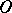-minimality), and the generalization of stability-theoretic techniques beyond the first-order context.

How to Cite This Entry:
Stability theory (in logic). Encyclopedia of Mathematics. URL: http://encyclopediaofmath.org/index.php?title=Stability_theory_(in_logic)&oldid=12117
This article was adapted from an original article by J.T. Baldwin (originator), which appeared in Encyclopedia of Mathematics - ISBN 1402006098. See original article